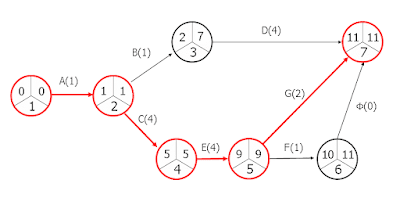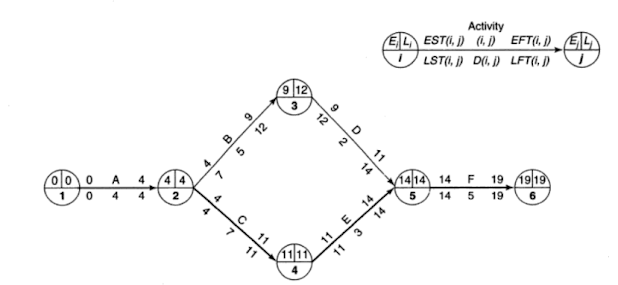# How to find Critical Path and Duration of a Construction Project?

The Critical Path Method (CPM) is essentially an algorithm followed in project scheduling for decision makings in construction projects. In this method, the start time, duration, and ending time of each activity involved in the project to figure out the activities that deserve more attention i.e. the critical activities.Image Credits: www.designingbuildings.co.uk

We will discuss the steps involved in developing a project network using the CPM technique, and conducting CPM analysis, followed by the determination of the critical path of a project.

### How to find the Critical Path in a Construction Project Schedule?

CPM network analysis follows a combination of critical and non-critical tasks. The steps involved in performing CPM network analysis are as below:

#### STEP 1: Identify and List the Construction Project Activities

All the project activities involved in a construction project are listed using the Work Breakdown Structure (WBS) concept. The items are listed as activities or tasks. These activities serve as the foundation for the rest of the CPM analysis. These items are the ones necessary to produce the project deliverables.

#### STEP 2: Identify the dependencies

Each activity may or may not be dependent on the preceding activity. For example, an activity "foundation" cannot be started before the activity "excavation". Hence, all the activities and their interdependencies must be properly studied.

#### STEP 3: Create the Network Diagram

There are certain planning techniques and terminologies involved in making a network for a construction project.
Consider the starting activity of a project as "A". It can be represented as A-O-A (Activity on Activity )or the A-O-N (Activity on Node) method. If we represent in the A-O-A method, we have the activity, A represented in the following way.

In the above figure,  activity 'A' is represented as A(1,2), where 1 and 2 are nodes of the activity. They do not have a high significance in network analysis. They are used to represent the start and finish of activity A. Generally an activity can be represented as A(i,j), where i and j are the nodes.

The figure shows a network 1-2-3-4-5-6. The duration of each activity is written below the arrow, down to the activity name as shown below. The network is developed based on its dependencies. The only data available is the duration of each activity, i.e. A (1,2) - 4 days, B (2,3)- 5 days, C (2,4) - 7 days, D(3,5)- 2 days, E (4,5) -3 days, F(5,6)- 5 days.

#### STEP 4: Determine Earliest and Latest Occurrence times of Each Construction Activity

Each activity will have start times ( Earliest start times (EST) & Latest Start times (LST)) and Finish Times ( Earliest Finish Time (EFT) and Latest Finish Times (LFT)). If so, the start times and finish times at respective nodes i and j are;Fig.5

#### STEP 5: Determine Float/Slack

Float or slack refers to the time or how much the task can be delayed without affecting the whole project schedule. It is the amount of flexibility given to a task. There are different types of float. In order to determine the critical path, we need to determine the total float.

Total Float = Latest Finish Time (LFT) - Earliest Finish Time (EFT) of an activity
or
= Latest Start Time (LST) - Earliest Start Time (EST) of an activity

Total Float TF = LST - EST = Li - Ei =  Lj - D(i,j) - Ei

TF = Li - Ei - D(i,j)

#### Step 6: Determination of Critical Activities and Critical Path

Activities in a network that has zero total floats are called critical activities. For critical activities, the EST=LST and EFT = LFT.

The path joining the critical activities in a network is called the critical path. The critical path is the longest path in the network. The time along the critical path determines the completion time of the project.

In figure.5, the total float is zero for the activities along, 1-2-4-5-6. Hence the critical path is 1-2-3-4-5-6 and the project completion time is 19 days. The critical path is marked in bolded line.